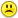+0

# Could somebody help me check this?

+5
1099
16Apr 21, 2015

#13
+10

Look at the step where fiora sets -1 + √3i to 3√(-1 + √3i)3

In the next step (-1 + √3i)3 is expanded, correctly, to get 8, but then the wrong cube root of 8 is taken.  Fiora says the cube root of 8 is 2.  If this were the right thing to do here then it would mean that  -1 + √3i equals 2, which would be a little unusual to say the least!

The fact is that, in the domain of complex numbers, there are three cube roots of 8, namely 2, -1 - √3i and -1 + √3i.  The only valid root here is the last one.  It isn't valid to jump from -1 + √3i to (-1 + √3i)3 and then jump back to a different root from the one you came from!

It's like saying -2 squared is 4, the square root of 4 is 2, so we can replace -2 with 2.

.

Apr 21, 2015

#1
0

i stand for sqrt -1 ......and i might not be here aftet a minute....

Apr 21, 2015
#2
0

Men, I had been working on this several days,recently figured this out....

Apr 21, 2015
#3
+5

I am going to sleep.See you everybody

Apr 21, 2015
#4
+5

Hi Fiora,  welcome to the forum.

Did I talk to you before and suggest that you join and that you take that icon?

It looks great :))

-------------------------------------------------

Edited: this was wrong

Apr 21, 2015
#5
+10

FWIW, I got the same answer as fiora.

# ☼☼☼

Apr 21, 2015
#6
+10

Sorry Badinage and fiora,  I think you are right - I was suffering from brain freeze

I have not looked from there on Badinage, is the rest of Fiora's work correct?

$$\\(-1+\sqrt3i)^3\\\\ =(\sqrt3i-1)^3\\\\ =[(\sqrt3i)^3]+[3(\sqrt3i)^2(-1)]+[3(\sqrt3i)(-1)^2]+[-1^3]\\\\ =[3\sqrt3*-i]+[-3(3*-1)]+[3(\sqrt3i)]+[-1]\\\\ =[-3\sqrt3i]++[3\sqrt3i]+[-1]\\\\ =++[-1]\\\\ =+8$$

Apr 21, 2015
#7
+10

Wolfram alpha doesn't agree with any of us.http://m.wolframalpha.com/input/?i=%28+%28-7+%2Bi21%28%283%29^0.5%29%29%2F2%29^1%2F3++&x=0&y=0&js=off

Apr 21, 2015
#8
+10

$$\\(1+\sqrt3i)^3\\\\ =(\sqrt3i+1)^3\\\\ =[(\sqrt3i)^3]+[3*(\sqrt3i)^2]+[3*(\sqrt3i)]+1\\\\ =(3\sqrt3*-i)+(3*3*-1)+(3*\sqrt3i)+1\\\\ =(-3\sqrt3i)+(-9)+(3\sqrt3i)+1\\\\ =-8$$

SO

$$\\\sqrt{ \frac{14*\sqrt{(1+3i)^3} }{2}}\\\\ =\sqrt{ \frac{14*\sqrt{-8} }{2}}\\\\ =\sqrt{ \frac{14*\sqrt{8}*\sqrt{-1} }{2}}\\\\ =\sqrt{ \frac{14*2*\sqrt{-1} }{2}}\\\\ =\sqrt{ 14\sqrt{-1} } \\\\$$

I have yet to consider   $$\sqrt{-1}$$

Does that look right or wrong to you Badinage?

Apr 21, 2015
#9
+5

I had a quick look at Wolfram|Alpha  and  I cannot tell whether it agrees with mine or not since mine is not finished :)

Apr 21, 2015
#10
+10

Now I have to consider

$$\\\sqrt{-1}\\ The first obvious answer is  -1+0i  \;\;since (-1)^3=-1\\ Now, there will be 3 roots\\ each will be 2\pi/3  radians apart. \\ So the other 2 will be \\ =cos(\pi/3)+isin(\pi/3)\qquad and \qquad cos(-\pi/3)+isin(-\pi/3)\\ =\qquad \frac{1}{2}+\frac{\sqrt3i}{2} \qquad \qquad \qquad and\qquad \qquad \frac{1}{2}-\frac{\sqrt3i}{2}\\ =\qquad \frac{1+\sqrt3i}{2} \qquad \qquad \qquad and\qquad\qquad\frac{1-\sqrt3i}{2}$$

NOW

$$\\\sqrt{-1}=-1,\qquad \frac{1+\sqrt3i}{2}, \qquad\frac{1-\sqrt3i}{2}\\ so\\ \sqrt{14\sqrt{-1}}\\\\ =\sqrt{14(-1)},\qquad \sqrt{14*\frac{1+\sqrt3i}{2}}, \qquad \sqrt{14*\frac{1-\sqrt3i}{2}}\\\\ =\sqrt{-14},\qquad \sqrt{7(1+\sqrt3i)}, \qquad \sqrt{7(1-\sqrt3i)}\\\\ Now WolframAlpha only gave 3 answers but here I am going to get 3 lots of 3 answers, perhaps each lot is the same as the other 3 lots?\\\\ \sqrt{-14}\\\\ =\sqrt{14}\sqrt{-1}\\\\ =-\sqrt{14},\qquad \sqrt{14}*\frac{1+\sqrt3i}{2}, \qquad\sqrt{14}*\frac{1-\sqrt3i}{2}\\\\$$

$$\\This approximates to\\\\ =-2.410,\qquad 2.410*\frac{1+\sqrt3i}{2}, \qquad2.410*\frac{1-\sqrt3i}{2}\\\\ =-2.410,\qquad 2.410*(0.5+\frac{\sqrt3i}{2}), \qquad2.410*(0.5-\frac{\sqrt3i}{2})\\\\ =-2.410,\qquad 1.205+2.087i, \qquad 1.205-2.087i\\\\$$

Badinage is right.   None of these answers agree with Wolfram|alphaApr 21, 2015
#11
+10

The best method for dealing with powers and roots of complex numbers, is to switch them to polar form and then to use De Moivre's theorem.

So here,

$$\displaystyle \frac{-7+21\sqrt{3}\imath}{2}=-\frac{7}{2}(1-3\sqrt{3}\imath)=-\frac{7}{2}\sqrt{28}\angle\tan^{-1}(-3\sqrt{3})$$

(ask for details if you need them)

$$\displaystyle = -7\sqrt{7}\angle 280.89339\deg.$$

Raise that to the power one third and you have,

$$(-7\sqrt{7}\angle(-280.89339+360k))^{1/3}$$

$$\displaystyle = -\sqrt{7}\angle(93.63113+120k), \quad k=0,1,2.$$

That gets you

$$\displaystyle k=0:\quad -\sqrt{7}\angle 93.63113 = -\sqrt{7}(\cos93.63113+\imath\sin93.63113)$$

$$\displaystyle =0.16752-2.64044\imath.$$

and the other two values of k,

$$\displaystyle 2.20291+1.46533\imath \;\text{ and } -2.37047+1.17511\imath \quad\text{respectively}.$$

Apr 21, 2015
#12
+5

I'd like someone to point out the flaw in fiora's working. I agree there exist more than one answer, but where is the error in fiora's calculation if the result obtained there is not one of the valid answers?

Apr 21, 2015
#13
+10

Look at the step where fiora sets -1 + √3i to 3√(-1 + √3i)3

In the next step (-1 + √3i)3 is expanded, correctly, to get 8, but then the wrong cube root of 8 is taken.  Fiora says the cube root of 8 is 2.  If this were the right thing to do here then it would mean that  -1 + √3i equals 2, which would be a little unusual to say the least!

The fact is that, in the domain of complex numbers, there are three cube roots of 8, namely 2, -1 - √3i and -1 + √3i.  The only valid root here is the last one.  It isn't valid to jump from -1 + √3i to (-1 + √3i)3 and then jump back to a different root from the one you came from!

It's like saying -2 squared is 4, the square root of 4 is 2, so we can replace -2 with 2.

.

Alan Apr 21, 2015
#14
+5

Thanks Bertie, I shall have to take time to look at your answer  :)

Thanks for that great explanation Alan, I had vagely thought there could be problems but your explanation clarified it for me.   :)

Apr 21, 2015
#15
0

Did you write this with your left or right foot?Apr 21, 2015
#16
+5

Thanks Alan..

Apr 21, 2015